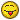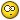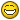# In theory, could this be done?

Had an idea for a tool I’d like to make but just thought I’d ask to see if it’s feasible before I delve into it.
I work with code a lot but I’ve never done any Lua and have no real knowledge of the Renoise API, yet…

Anyway, I thought it would be cool to make a tool that simply replaces whatever sample is in the currently selected instrument and sample slot with a file chosen at random from a given folder (and their subfolders).

I have a huge folder tree full of drum hits, snippets, textures, field recordings - I’m sure we all have a similar setup, and I think being able to just pull up something from the archive completely at random could be inspiring, like a creative chance card or Oblique Strategy.

Here’s what I’m imagining it would need to do:

• Let the user select a folder to use as the sample pool
• Scan the folder and subfolders for usable sample files
• Store the file paths, filesizes, and preferably durations in seconds in an array
• Allow the user to select a minimum and maximum filesize and/or duration, making a copy of the original array with those outside of the parameters excluded after pressing an ‘apply’ button
• When the ‘go’ button is pressed, clear the currently selected sample slot and replace with a random choice from the pool

Is all of this possible with the Renoise API?

I can’t say if this is possible, but +1 for the idea!

What I sometimes do now in windows explorer is search my complete sample folder on a specific word, and use all results with the chosen word in its name in Renoise through drag & drop.

I would suggest you research first for yourself: http://code.google.com/p/xrnx/source/browse/trunk#trunk/Documentation

I’m sure you can create a file/directory browser using the GUI-API.
If you ask me, entering a path in an entry would be enough.

See the Lua.Standard.API at http://code.google.com/p/xrnx/source/browse/trunk/Documentation/Lua.Standard.API.lua

Durations could be tricky, I’m unsure that the API supports this.

Sounds like a more or less trivial GUI. Shuffling around data in arrays is
as easy as in other languages.

I don’t know if you can access the “currently selected sample slot”. The most
accessible part of Renoise from the API is the song-data itself, but not
the other GUI elements so much.

Generally I like the idea. But maybe writing a program which is not as tightly
integrated into Renoise would be more scalable. Where you enter the length/duration
of the samples you seek, and press the “generate random list” button and
the found/selected samples are copied into a separate directory. That directory
could then be accessed from the Renoise sample selector.

In practice this is pretty much possible.
sample time is pretty hard to calculate before even loading, but you can get the filesize using the io.stat()
Current selected sample slot is also achievable. Multiselection won’t work though.
But i guess one could build a GUI to add random samples up to the selected slot or from the selected slot or just only into the selected slot.

Here is at least a quick snippet to enumerate the files in folders and subfolders. The generic Lua examples that google pops up with, lean on some lfs library which Renoise doesn’t use, but it isn’t necessary either.
It is just a start, i hope it makes someone else enthusiastic to attempt it…

``````

local PATH_SEPARATOR = "/"

if (os.platform() == "WINDOWS") then
PATH_SEPARATOR = "\\"
end
local source = "your/path/to/sample/files/"

--Get recursive folder tree using io.dirnames...
local function get_folder_tree(root_path)
local root_tree = os.dirnames(root_path)
local i = 1

while i <= #root_tree do
local sub_tree = os.dirnames(root_path..root_tree[i])

for t = 1, #sub_tree do
root_tree[#root_tree + 1] = root_tree[i]..PATH_SEPARATOR..sub_tree[t]
end
i = i + 1
end

return root_tree
end

local sample_folders = get_folder_tree(source)

local sample_files = {}

for _ = 1,#sample_folders do
-- print("--->"..sample_folders[_])
local full_path = source..sample_folders[_]
local sub_folder_files = os.filenames(full_path)

for t = 1, #sub_folder_files do
local str = sample_folders[_]..PATH_SEPARATOR..sub_folder_files[t]
str = str:gsub("\\","/")
sample_files[#sample_files + 1] = str
end

end

``````

io.stat details (documentation\Lua.Standard.API.lua)

local cur_sample = renoise.song().selected_sample_index
local cur_instrument = renoise.song().selected_instrument_index

Randomize function

``````

function randomize(tstart, tend, buffer, compare)
local number = tostring(os.clock())
local approved = false
if tstart == 0 then
tstart = 1
end
if tend < tstart then
tend = tstart
end
if string.find(number,"%.") ~= nil then
number = string.sub(number, string.find(number,"%.")+1)
end

math.randomseed( tonumber(number))
number = number + math.random(1, 30)
math.randomseed( tonumber(number))
math.random(tstart, tend); math.random(tstart, tend); math.random(tstart, tend)
local result = math.random(tstart, tend)
return result
end

``````

I’m not going to add more else the tool is more or less literally doneWeird function you got there. What does it do more than math.random (tstart, tend) does?
I don’t think that setting a new random seed would have much effect on the user experience. Also, buffer and compare are not used.

What you want is to shuffle your array of fitting samples. For that I
suggest the more or less simple algorithm of Knuth shuffle (aka fisher-yates-shuffle):

(I found this code via google at http://www.gammon.com.au/forum/?id=9908 )

``````
function shuffleArray(array)
local arrayCount = #array
for i = arrayCount, 2, -1 do
local j = math.random(1, i)
array[i], array[j] = array[j], array[i]
end
return array
end

``````

buffer and compare can indeed be removed. They were tables with numbers, I removed the routines that sorted those tables (because it was a function specific feature) to be able to use the function global wise in the tool.
And yeah, it doesn’t supposed to be that complicated, but for as far as i could recall, math.random didn’t really returned a true random figure. As a matter of fact, it was favoring a specific table of figures from which it picked a number and then restarted from scratch picking the same numbers. E.g. if i asked him to return a figure between 1 and 100, it would return consequently either 5,27,43 and 75, but nothing else, just one of those four numbers. (just an example sequence, it was not exactly those numbers) . I was frowning my eyebrows when i figured that out.
I can promise you, i did started that simple.

But maybe somebody can put that up to the test. At least there was something very weird with the math-random function and the seed generation routine.

Well, math.random is a pseudo random number generator (PRNG), so that is to be
expected. I always thought that maybe renoise would seed it internally with the
“current time” at startup - but thats probably not the case. When I was
experimenting with my Clip Arranger tool, which relies heavily on
randomization, I never experienced the pseudoness to be a problem. If it is a
problem, you usually seed the current time into it at startup, and not each
time you need a random number, because that would more or less defeat the
purpose of a PRNG - because then you could also just use a counter and the
current time and add them together somehow. Actually, thats almost what the
PRNG does, just that randomize() is resetting the internal state of
math.random() each time it reseeds the PRNG, so the PRNG is degraded into not
much more than a glorified number-obfuscation-function.

In fact, if you call your function multiple times in a row you will
often/always get the same output with your randomize() function (depending on
the precision of os.clock ()):

``````
function randomize(tstart, tend, buffer, compare)
local number = tostring(os.clock())
local approved = false
if tstart == 0 then
tstart = 1
end
if tend < tstart then
tend = tstart
end
if string.find(number,"%.") ~= nil then
number = string.sub(number, string.find(number,"%.")+1)
end

math.randomseed( tonumber(number))
number = number + math.random(1, 30)
math.randomseed( tonumber(number))
math.random(tstart, tend); math.random(tstart, tend); math.random(tstart, tend)
local result = math.random(tstart, tend)
return result
end

print ("randomize output:");
for v = 0, 10, 1 do
print (randomize (1, 100))
end

print ("math.random output with proper seed:");
math.randomseed (os.time ());
for v = 0, 10, 1 do
print (math.random (1, 100))
end

``````

If you run that script with lua directly you will notice that it always outputs
69 from your randomize() output:

``````
# lua -l math rnglua.lua
randomize output:
69
69
69
69
69
69
69
69
69
69
69
math.random output with proper seed:
94
82
35
44
11
1
68
64
41
58
45

``````

ALWAYS, because os.clock () will always return 0 for that tiny script. In
renoise, with other lua code running inbetween, that is of course another
story, but it still defeats the pseudo-randomness of the PRNG. The second loop
shows how the PRNG is should actually be used. The script will output the same
sequence of numbers only if you call it within the same second. But in either
case,it will generate a list of (pseudo) random numbers.

Not quite, os.clock() always returns a number, but if the time-span is really very small, os.clock() will then return the same number which i actually didn’t expected it would do, because i suspected the Lua engine would be slow enough to make it concoct a new seed for the new turn.

I now see the error (and how much instructions lua can perform per millisecond) of the routine…
My purpose is simply not working with the same seed if possible and i specially choosen os.clock() because it provides me more figures with larger differences (in the same time span) than os.time().

So this does work a lot better:

``````

random_seed = nil

function randomize(tstart, tend)
if (os.clock()*1000) ~= random_seed then
random_seed = os.clock()*1000
math.randomseed(random_seed)
end

if tstart == 0 then
tstart = 1
end

if tend < tstart then
tend = tstart
end

return math.random(tstart, tend)
end

function random_test()
print ("randomize output:");
for v = 0, 1000, 1 do
print (randomize (1, 100))
end

print ("math.random output with proper seed:");
math.randomseed (os.time ());
print("time:"..os.time())

for v = 0, 10, 1 do
print (math.random (1, 100))
end
end

``````

Thanks for the refreshment class.

I like that.

So in short, the whole idea is possible especially if you regard the ‘sample duration’ part as a “should have”… For that you gotta go and implement a reader for the .wav header, .flac header, .mp3 header, etc etc.
The other thing I would worry about is whether to save the database in the “preferences” space (as it can contain tables but not sure if it’s optimised for ~1000 entry tables or larger) or in another format.

It’s a good idea.

@elmex: your first reply is really unnecessary there, it gives no real info whatsoever and I understand wanting to encourage others to research for themselves but that reply could be really discouraging actually. OP asks the question what’s possible, he’s not (yet) asking anybody to make it for him. You are not helping anybody with that post.

You could’ve taken this from the docs instead:

``````
renoise.app():prompt_for_path(dialog_title)

``````

I’m thinking right now, the proper tool should actually init/update the database when not playing, and load every sample once to check for the sample format and duration. Then maybe add some user configurable properties like type (loop or one shot), category (possibly derived from the folder it is in, but multiple categories should be possible).

If I had known that function, I would certainly have supplied it. But my
knowledge limited, and so is the time to read into API docs for someone else’s
problem. So should I say nothing in the future instead?

PS: Giving links to API documentation is helpful in my opinion. If there is not
enough motivation to do some document reading, then there certainly will be not
enough motivation to produce a piece of software.

Sounds like a fun tool. I’d imagine this would be great for instance with the adventure kid waveform pack! A fresh sound always one click away.

Thanks for the info everyone, it was all helpful.
Seems this is totally possible with the API and shouldn’t be that hard either, once I get used to ending lines without a semicolonSame here, every time I come back to Lua@elmex, my apologies, I was in some way convinced that your first reply consisted of mostly indefinite, unsure, showstopper answers, I definitely didn’t read it well enough.

What’s everybody’s idea about a database/table type? Just a bigass lua table saved into preferences.xml?